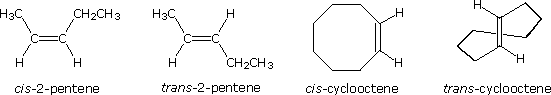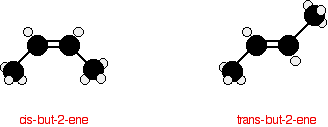# 8.2: Alkenes—The Products of Elimination Reactions

$$\newcommand{\vecs}{\overset { \rightharpoonup} {\mathbf{#1}} }$$ $$\newcommand{\vecd}{\overset{-\!-\!\rightharpoonup}{\vphantom{a}\smash {#1}}}$$$$\newcommand{\id}{\mathrm{id}}$$ $$\newcommand{\Span}{\mathrm{span}}$$ $$\newcommand{\kernel}{\mathrm{null}\,}$$ $$\newcommand{\range}{\mathrm{range}\,}$$ $$\newcommand{\RealPart}{\mathrm{Re}}$$ $$\newcommand{\ImaginaryPart}{\mathrm{Im}}$$ $$\newcommand{\Argument}{\mathrm{Arg}}$$ $$\newcommand{\norm}{\| #1 \|}$$ $$\newcommand{\inner}{\langle #1, #2 \rangle}$$ $$\newcommand{\Span}{\mathrm{span}}$$ $$\newcommand{\id}{\mathrm{id}}$$ $$\newcommand{\Span}{\mathrm{span}}$$ $$\newcommand{\kernel}{\mathrm{null}\,}$$ $$\newcommand{\range}{\mathrm{range}\,}$$ $$\newcommand{\RealPart}{\mathrm{Re}}$$ $$\newcommand{\ImaginaryPart}{\mathrm{Im}}$$ $$\newcommand{\Argument}{\mathrm{Arg}}$$ $$\newcommand{\norm}{\| #1 \|}$$ $$\newcommand{\inner}{\langle #1, #2 \rangle}$$ $$\newcommand{\Span}{\mathrm{span}}$$

Ethene is the formal IUPAC name for H2C=CH2, but it also goes by a common name: Ethylene. The name Ethylene is used because it is like an ethyl group ($$CH_2CH_3$$) but there is a double bond between the two carbon atoms in it. Ethene has the formula $$C_2H_4$$ and is the simplest alkene because it has the fewest carbons (two) necessary for a carbon-carbon double bond.

## Introduction

Bonding in carbon is covalent, containing either sigma or $$\pi$$ bonds. Carbon can make single, double, or triple bonds. The number of bonds it makes determines the structure. With four single bonds, carbon has a tetrahedral structure, while with one double bond it's structure is trigonal planar, and with a triple bond it has a linear structure.

A solitary carbon atom has four electrons, two in the 2s orbital, and one in each of the 2$$p_x$$ and 2$$p_y$$ orbitals, leaving the $$2p_z$$ orbital empty. A single carbon atom can make up to four bonds, but by looking at its electron configuration this would not be possible because there are only two electrons available to bond with. The other two are in a lone pair state, making them much less reactive to another electron that is by itself. Well it is, in order to make the four bonds, the carbon atom promotes one of the 2s electrons into the empty $$2p_z$$ orbital, leaving the carbon with four unpaired electrons allowing it to now form four bonds. The electron is not promoted spontaneously. It becomes promoted when a photon of light with the correct wavelength hits the carbon atom. When this photon hits the carbon atom it gives the atom enough energy to promote one of the lone pair electrons to the $$2p_z$$ orbital.## Sigma and Pi Bonds

All the bonds in Ethene are covalent, meaning that they are all formed by two adjacent atoms sharing their valence electrons. As opposed to ionic bonds which hold atoms together through the attraction of two ions of opposite charges.

Sigma bonds are created when there is overlap of similar orbitals, orbitals that are aligned along the inter-nuclear axis. Common sigma bonds are $$s+s$$, $$p_z+p_z$$ and $$s+p_z$$, $$z$$ is the axis of the bond on the xyz-plane of the atom.

$$\pi$$ bonds are created when there is adequate overlap of similar, adjacent $$p$$ orbitals, such as $$p_x$$+$$p_x$$ and $$p_y$$+$$p_y$$. Each p orbital has two lobes, one usually indicated by a + and the other indicated by a - (sometimes one may be shaded while the other is not). This + and - (shaded, not shaded) are only meant to indicate the opposite phase $$\phi$$ the wave functions, they do not indicate any type of electrical charge. For a $$\pi$$ bond to form both lobes of the $$p$$ orbital must overlap, + with + and - with -. When a + lobe overlaps with a - lobe this creates an anti-bonding orbital interaction which is much higher in energy, and therefore not a desirable interaction.

Usually there can be no $$\pi$$ bonds between two atoms without having at least one sigma bond present first. But there are special cases such as dicarbon ($$C_2$$) where the central bond is a $$\pi$$ bond not a sigma bond, but in cases like these the two atoms want to have as much orbital overlap as possible so the bond lengths between the atoms are smaller than what is normally expected.

The $$\pi$$ bond in ethene is weak compared to the sigma bond between the two carbons. This weakness makes the $$\pi$$ bond and the overall molecule a site of comparatively high chemical reactivity to an array of different substances. This is due to the high electron density in the $$\pi$$ bond, and because it is a weak bond with high electron density the $$\pi$$ bond will easily break in order to form two separate sigma bonds. Sites such as these are referred to as functional groups or functionalities. These groups have characteristic properties and they control the reactivity of the molecule as a whole. How these functional groups and other reactants form various products are an important concept in organic chemistry.

## Orbital Bonding in Ethene

Ethene is made up of four 1s1 Hydrogen atoms and two 2s2 2$$p_x$$1 2$$p_y$$2 carbon atoms. These carbon atoms already have four electrons, but they each want to get four more so that they have a full eight in the valence shell. Having eight valence electrons around carbon gives the atom itself the same electron configuration as neon, a noble gas. Carbon wants to have the same configuration as Neon because when it has eight valence electrons carbon is at its most stable, lowest energy state, it has all of the electrons that it wants, so it is no longer reactive.

## Structure of Ethene

Ethene is not a very complicated molecule. It contains two carbon atoms that are double bonded to each other, with each of these atoms also bonded to two Hydrogen atoms.This forms a total of three bonds to each carbon atom, giving them an $$sp^2$$ hybridization. Since the carbon atom is forming three sigma bonds instead of the four that it can, it only needs to hybridize three of its outer orbitals, instead of four. It does this by using the $$2s$$ electron and two of the $$2p$$ electrons, leaving the other unchanged. This new orbital is called an $$sp^2$$ hybrid because that's exactly what it is, it is made from one s orbital and two p orbitals.When atoms are an $$sp^2$$ hybrid they have a trigonal planar structure. These structures are very similar to a 'peace' sign, there is a central atom with three atoms around it, all on one plane. Trigonal planar molecules have an ideal bond angle of 120° on each side.The H-C-H bond angle is 117°, which is very close to the ideal 120° of a carbon with $$sp^2$$ hybridization. The other two angles (H-C=C) are both 121.5°.

## Rigidity in Ethene

There is rigidity in the Ethene molecule due to the double-bonded carbons. In Ethane there are two carbons that share a single bond, this allows the two Methyl groups to rotate with respect to each other. These different conformations result in higher and lower energy forms of Ethane. In Ethene there is no free rotation about the carbon-carbon sigma bond. There is no rotation because there is also a $$\pi$$ bond along with the sigma bond between the two carbons. A $$\pi$$ bond is only formed when there is adequate overlap between both top and bottom p-orbitals. In order for there to be free rotation the p-orbitals would have to go through a phase where they are 90° from each other, which would break the $$\pi$$ bond because there would be no overlap. Since the $$\pi$$ bond is essential to the structure of Ethene it must not break, so there can be not free rotation about the carbon-carbon sigma bond.

## Configurational Stereoisomers of Alkenes

The carbon-carbon double bond is formed between two sp2 hybridized carbons, and consists of two occupied molecular orbitals, a sigma orbital and a pi orbital. Rotation of the end groups of a double bond relative to each other destroys the p-orbital overlap that creates the pi orbital or bond. Because the pi bond has a bond energy of roughly 60 kcal/mole, this resistance to rotation stabilizes the planar configuration of this functional group. As a result, certain disubstituted alkenes may exist as a pair of configurational stereoisomers, often designated cis and trans. The essential requirement for this stereoisomerism is that each carbon of the double bond must have two different substituent groups (one may be hydrogen). This is illustrated by the following general formulas. In the first example, the left-hand double bond carbon has two identical substituents (A) so stereoisomerism about the double bond is not possible (reversing substituents on the right-hand carbon gives the same configuration). In the next two examples, each double bond carbon atom has two different substituent groups and stereoisomerism exists, regardless of whether the two substituents on one carbon are the same as those on the other.Some examples of this configurational stereoisomerism (sometimes called geometric isomerism) are shown below. Note that cycloalkenes smaller than eight carbons cannot exist in a stable trans configuration due to ring strain. A similar restriction holds against cycloalkynes smaller than ten carbons. Since alkynes are linear, there is no stereoisomerism associated with the carbon-carbon triple bond.## Geometric (cis-trans) isomerism

The carbon-carbon double bond doesn't allow any rotation about it. That means that it is possible to have the CH3 groups on either end of the molecule locked either on one side of the molecule or opposite each other.

These are called cis-but-2-ene (where the groups are on the same side) or trans-but-2-ene (where they are on opposite sides).Cis-but-2-ene is also known as (Z)-but-2-ene; trans-but-2-ene is also known as (E)-but-2-ene. For an explanation of the two ways of naming these two compounds, follow the link in the box below.

## Stability of Alkenes

The stability of alkene can be determined by measuring the amount of energy associated with the hydrogenation of the molecule. Since the double bond is breaking in this reaction, the energy released in hydrogenation is proportional to the energy in the double bond of the molecule. This is a useful tool because heats of hydrogenation can be measured very accurately. The ?H° is usually around -30 kcal/mol for alkenes. Stability is simply a measure of energy. Lower energy molecules are more stable than higher energy molecules. More substituted alkenes are more stable than less substituted ones due to hyperconjugation. They have a lower heat of hydrogenation. The following illustrates stability of alkenes with various substituents:In disubstituted alkenes, trans isomers are more stable than cis isomers due to steric hindrance. Also, internal alkenes are more stable than terminal ones. See the following isomers of butene:Trans-2-butene is the most stable because it has the lowest heat of hydrogenation.

Note: In cycloalkenes smaller than cyclooctene, the cis isomers are more stable than the trans as a result of ring strain.

## Contributors

This page titled 8.2: Alkenes—The Products of Elimination Reactions is shared under a not declared license and was authored, remixed, and/or curated by Layne Morsch.